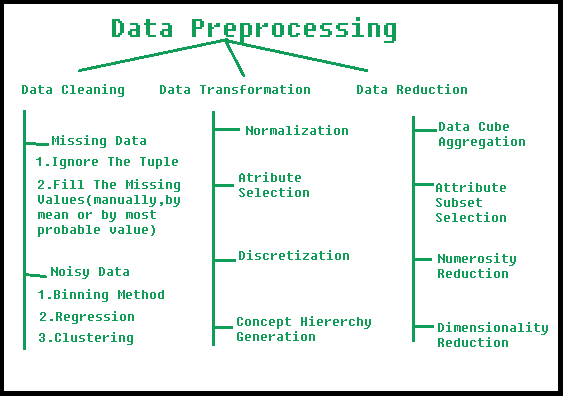# Data Preprocessing in Data Mining

• Difficulty Level : Medium
• Last Updated : 29 Jun, 2021

Preprocessing in Data Mining:
Data preprocessing is a data mining technique which is used to transform the raw data in a useful and efficient format.Steps Involved in Data Preprocessing:

1. Data Cleaning:
The data can have many irrelevant and missing parts. To handle this part, data cleaning is done. It involves handling of missing data, noisy data etc.

• (a). Missing Data:
This situation arises when some data is missing in the data. It can be handled in various ways.
Some of them are:
1. Ignore the tuples:
This approach is suitable only when the dataset we have is quite large and multiple values are missing within a tuple.

2. Fill the Missing values:
There are various ways to do this task. You can choose to fill the missing values manually, by attribute mean or the most probable value.

• (b). Noisy Data:
Noisy data is a meaningless data that can’t be interpreted by machines.It can be generated due to faulty data collection, data entry errors etc. It can be handled in following ways :
1. Binning Method:
This method works on sorted data in order to smooth it. The whole data is divided into segments of equal size and then various methods are performed to complete the task. Each segmented is handled separately. One can replace all data in a segment by its mean or boundary values can be used to complete the task.

2. Regression:
Here data can be made smooth by fitting it to a regression function.The regression used may be linear (having one independent variable) or multiple (having multiple independent variables).

3. Clustering:
This approach groups the similar data in a cluster. The outliers may be undetected or it will fall outside the clusters.

2. Data Transformation:
This step is taken in order to transform the data in appropriate forms suitable for mining process. This involves following ways:

1. Normalization:
It is done in order to scale the data values in a specified range (-1.0 to 1.0 or 0.0 to 1.0)

2. Attribute Selection:
In this strategy, new attributes are constructed from the given set of attributes to help the mining process.

3. Discretization:
This is done to replace the raw values of numeric attribute by interval levels or conceptual levels.

4. Concept Hierarchy Generation:
Here attributes are converted from lower level to higher level in hierarchy. For Example-The attribute “city” can be converted to “country”.

3. Data Reduction:
Since data mining is a technique that is used to handle huge amount of data. While working with huge volume of data, analysis became harder in such cases. In order to get rid of this, we uses data reduction technique. It aims to increase the storage efficiency and reduce data storage and analysis costs.

The various steps to data reduction are:

1. Data Cube Aggregation:
Aggregation operation is applied to data for the construction of the data cube.

2. Attribute Subset Selection:
The highly relevant attributes should be used, rest all can be discarded. For performing attribute selection, one can use level of significance and p- value of the attribute.the attribute having p-value greater than significance level can be discarded.

3. Numerosity Reduction:
This enable to store the model of data instead of whole data, for example: Regression Models.

4. Dimensionality Reduction:
This reduce the size of data by encoding mechanisms.It can be lossy or lossless. If after reconstruction from compressed data, original data can be retrieved, such reduction are called lossless reduction else it is called lossy reduction. The two effective methods of dimensionality reduction are:Wavelet transforms and PCA (Principal Component Analysis).

My Personal Notes arrow_drop_up
Recommended Articles
Page :